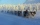# Melting point

The melting point of mercury is -36°F and its boiling point is 672°F. What is the difference between the boiling point and the melting point?

Result

t =  708 °F

#### Solution:Leave us a comment of example and its solution (i.e. if it is still somewhat unclear...):

Showing 0 comments:Be the first to comment!## Next similar examples:

1. On SundayOn Sunday the temperature reached a high of 38° above 0. That night it dropped to 23° below 0. What is the difference between the high and low temperatures for Sunday?
2. The lowestThe lowest temperature on Thursday was 20°C. The lowest temperature on Saturday was 12°C. What was the difference between the lowest temperatures?
3. Difference in temperaturesThe temperature in the number line show minus 15 degree celsius and 8 degree celsius. What is the different between the temperatures?
4. 8 degrees8 degrees Celsius it cools down by 9 degrees Celsius. What is the resultant temperature?
5. LaboratoryMang Elio went to his laboratory at 7:00am And recorded that the temperature was 11.07°C. At lunchtime, the temperature rose by 27.9°C but dropped by 8.05°C at 5:00 PM. What was the temperature at 5:00 PM?
6. OpheliaOphelia recorded the temperature of a cold store every two hours. 1. At 6am it was -4°C and at 8am it was -1°C. By how much did the temperature rise? 2. The temperature went up by 5°C in the next two hours. .What was the temperature at 10am?
7. NoontimeIn the morning temperature was 110°F. By noontime it has gone up by 15°F. What was the noon temperature. (Give your answer in °F)
8. Temperature increaseIf the temperature at 9:am is 50 degrees. What is the temperature at 5:00pm if the temperature increases 4 degrees Fahrenheit each hour?
9. Degrees 2The temperature was 3°F and falls four degrees Fahrenheit. What is actually temperature?
10. Change in temperatureStarting temperature is 21°C, the highest temperature is 32°C. What is the change in temperature?
11. The temperartureThe temperarture is 0 degrees. It drops 5 degrees after 1 hour and then drops 3 degrees more the next hour. What is the temperature after 6 hours?
12. Round it0.728 round to units, tenths, hundredths.
13. Bitoo and ReenaBitoo ate 3/5 part of an apple and the remaining part was eaten by his sister Reena. How much part of an apple did Renna eat? Who had the larger share? By how much?
14. Cupcakes 2Susi has 25 cupcakes. She gives 4/5. How much does she have left?
15. Unknown number 11That number increased by three equals three times itself?
16. DisjointHow many elements have union and intersection of two disjoint sets when the first have 1 and secodn 8 elements.
17. Pizza 4Marcus ate half pizza on monday night. He than ate one third of the remaining pizza on Tuesday. Which of the following expressions show how much pizza marcus ate in total?